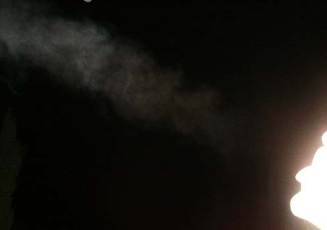## Condensation of your exhaled breathCondensation of Exhaled Breath (the temperature was around 11°C and the RH was about 90%). Note that the water vapor begins to condense only after it mixed with enough outside air, such that it could reach a RH of 100%, when the air cooled to about 32°C.
Ever wondered why and when does your exhaled breath condense? The simple answer is of course that as your breath mixes with the cold outside air, the relative humidity (RH) increases. If it can reach 100%, then breath condensation will commence.

This can be calculated with the breath condensation calculator.

If you're interested, here is the actual calculation. As the air mixes with the outside air, its state can be described using different combinations of three variables that are a function of the amount of "mixing" with the outside air. For example, we can use the air's pressure, temperature and relative humidity. Of course, some choices are wiser than others. Using the pressure, for example, is smart, since the pressure remains constant as the breath mixes with the outside air. Using the RH humidity, is well, not so smart, this is because the relative humidity depends on the temperature, since the saturation pressure of warmer vapor is higher (i.e., a given RH at one temperature implies a different amount of water content as the same RH at a different temperature).

A good choice for working variables are the pressure (p), the water content (g, i.e., how many grams of water per kg of air) and the enthalpy (which is the relevant free energy as described below). We describe these variables using the mixing ratio which is the ratio between the outside air mass to the total mass in the mixture. Thus, as the air is exhaled, its mixing ratio $f$ is 0, while it reaches 1 when the exhaled air has mixed with a large amount of outside air.

The variables we use are:
• The pressure $p$ of the mixed gas, which remains constant. That's easy.
• If the water content (gr water per kg of air) in the exhaled air is $g_0$ and the outside air $g_1$, then the total water content of the mixed gas is $g = (1-f) g_0 + f g_1$. This is because the total amount of water remains constant. To calculate $g$, we use the approximate relations that: $$g/[gr/kg] = 6.2 10^{-3} p_{w}/[Pa],$$ where the water vapor pressure is: $$p_{w}/[Pa] = RH \times 610.8 \exp \left( { 17.2694 T_C \over T_C + 238.3^\circ} \right) .$$ Here, $T_C$ is the temperature in °C.
Thus, given the temperature and RH, the water content can be calculated.
• The last variable to use is the enthalpy of the system. Because the mixing takes place under constant pressure, it is the total enthalpy $H = U + PV$ and not the internal energy $U$ which is conserved. This can be seen using the first law of Thermodynamics (which is essentially conservation of energy):
$$dH = dQ -dW + d(PV) = dQ - pdV + p dV + V dp = dQ + V dp = 0$$ in a process which is both adiabatic $(dQ=0)$ and under constant pressure $(dp=0)$. We use an approximation for the enthalpy, which is: $${h \over [kJ/kg]} \approx (1.007 T_C-0.026)+{g\over [gr/kg]} \times (2.501+0.00184 T_C) .$$ If the water vapor actually started to condense, we have to consider the fact that the enthalpy of the condensed water is lower by the heat of vaporation. If we separate the water content to the vapor and condensed parts, $g=g_v+g_c$, we have: $${h \over [kJ/kg]} \approx (1.007 T_C-0.026)+{g_v\over [gr/kg]} \times (2.501+0.00184 T_C)$$ $$+ {g_c\over [gr/kg]} \times 0.00419 T_C .$$ For a mixture, we have $h = (1-f) h_0 + f h_1$.

Parameters of the exhaled breath
A common misconception is that exhaled air is 100% saturated at 37°C. If this were the case, we would have seen condensation under any condition with outside air colder than 37°C. Obviously this is not the case! The reason is not that the air inside the lungs cannot reach equilibrium with the humid surfaces of the alveoli, in fact, because of the huge total surface area, equilibrium is reach immediately, however, the equilibrium is between the air and the saline solution composing us. Moreover, the saturation vapor pressure above a saline solution is lower than over pure water. (This is because some of the water molecules in the saline solution are bound to the salt ions and therefore cannot evaporate). Thus, air leaving our mouths is only about 35°C and 95% humid.

Using these relations, we can calculate the water content and enthalpy of the exhaled air and outside air, and using the mixture relations, obtain $g(f)$ and $h(f)$. By inverting the relations above, we can thus obtain $T(f)$ and $RH(f)$. If for any mixing ratio, we obtain RH>100%, we necessarily get condensation. The smaller the initial mixing ratio for condensation, the closer to our mouth it begins. Also, larger final mixing ratio correspond to longer durations until the mixed exhaled air dilutes enough for the condensation to evaporate again. Moreover, higher maximum condensed water content implies a thicker condensation.

Here is the actual exhaled breath condensation calculator.

## Blogroll

Sensible Climate Physics and more Other